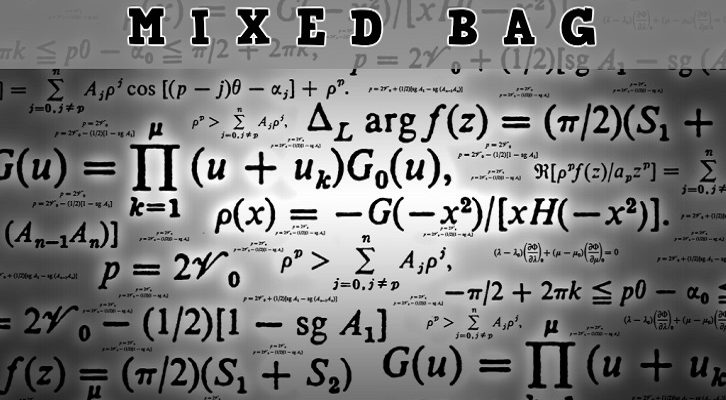# Solutions – Mixed bag

Have given solutions to the questions posted here

1. Average of 6 distinct positive integers is 33. The median of the three largest numbers is 43. What is the difference between the highest and lowest possible median of the 6 numbers?

Let a, b, c, d, e, f be the six numbers in ascending order

e = 43; a + b + c + d + e + f = 198

Median of 6 integers = (c +d)/2

(c +d)/2 is maximum when c and d are maximum since e = 43, dmax = 42 and cmax = 41

(c +d)/2 is minimum when c and d are minimum amin = 1, bmin = 2, cmin = 3 dmin = 4

Therefore, = (c +d)/2 min = 3.5

Max – min = 41.5 – 3.5 = 38

2. In class A, the ratio of boys to girls is 2:3. In class B the ratio of boys to girls is 4 : 5. If the ratio of boys to girls in both classes put together is 3 : 4, what is the ratio of number of girls in class A to number of girls in class B?

Let us assume class A has 2x boys and 3x girls, class B has 4y boys and 5y girls.

(2x + 4y)/(3x + 5y) = ¾

8x + 16y = 9x + 15y

x = y

Reqd ratio = 3x/5y (since x = y) = 3/5

3. N is an 80-digit positive integer (in the decimal scale). All digits except the 44th digit (from the left) are 2. If N is divisible by 13, find the 26th digit.

To begin with, the question should read “find the 44th digit”.

Any number of the form abcabc is a multiple of 1001. 1001 is 7 * 11 * 13. So, any number of the form abcabc is a multiple of 13.

So, a number comprising 42 2’s would be a multiple of 13, so would a number comprising 36 2’s. So, in effect, we are left with a two digit number 2a, where a is the 44th digit. 26 is a multiple of 13, so the 44th digit should be 6.

4. A page is torn from a novel. The sum of the remaining digits is 10000. What is the sum of the two page-numbers on the torn page of this novel?

n(n+1)/2 should be nearby 10,000, so n(n+1) is somewhere near 20,000. So n should be around sqrt(20,000) about 141. Try 142

142*143 = 10153

141 *142 = 10011

So, the missing pages are either 76 and 77 or 5 & 6